Courses

# Analysis of CE Amplifier Electrical Engineering (EE) Notes | EduRev

## Electronic Devices

Created by: Cstoppers Instructors

## Electrical Engineering (EE) : Analysis of CE Amplifier Electrical Engineering (EE) Notes | EduRev

The document Analysis of CE Amplifier Electrical Engineering (EE) Notes | EduRev is a part of the Electrical Engineering (EE) Course Electronic Devices.
All you need of Electrical Engineering (EE) at this link: Electrical Engineering (EE)

Analysis of CE amplifier Voltage gain:
To find the voltage gain, consider an unloaded CE amplifier. The ac equivalent circuit is shown in fig. 3. The transistor can be replaced by its collector equivalent model i.e. a current source and emitter diode which offers ac resistance r'e.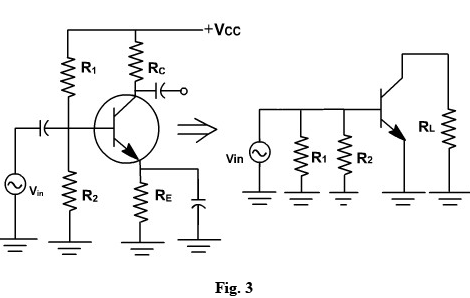The input voltage appears directly across the emitter diode.

Therefore emitter current, ie = Vin / r'e.

Since, collector current approximately equals emitter current and iC = ie and vout = - ie RC (The minus sign is used here to indicate phase inversion)

Further vout = - (Vin RC) / r'e

Therefore voltage gain A = vout / vin = -RC / r'e

The ac source driving an amplifier has to supply alternating current to the amplifier. The input impedance of an amplifier determines how much current the amplifier takes from the ac source.

In a normal frequency range of an amplifier, where all capacitors look like ac shorts and other reactances are negligible, the ac input impedance is defined as-

zin= vin/ iin

Where vin, iin are peak to peak values or rms values

The impedance looking directly into the base is symbolized zin (base) and is given by

Z in(base) = vin / ib ,

Since,vin = ie r'e

zin (base) =  r'e.

From the ac equivalent circuit, the input impedance zin is the parallel combination of R1 , R2 and r'e.

Zin = R1 || R2 ||  r'e

The Thevenin voltage appearing at the output is

vout = A vin

The Thevenin impedance is the parallel combination of RC and the internal impedance of the current source. The collector current source is an ideal source, therefore it has an infinite internal impedance.

zout = RC.

The simplified ac equivalent circuit is shown in fig. 4.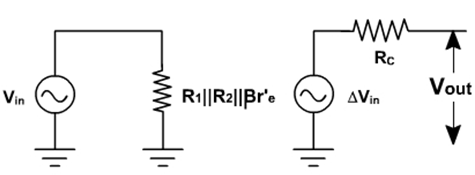fig. 4

Analysis of CE amplifier Example-1:

Select R1 and R2 for maximum output voltage swing in the circuit shown in fig. 5.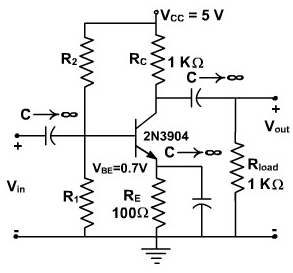Fig. 5

Solution:

We first determine ICQ for the circuit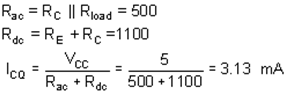For maximum swing,

VCC= 2 VCEQ

The quiescent value for VCE is the given by

VCEQ= (3.13 mA) (500 W ) = 1.56 V

The intersection of the ac load line on the VCE axis is VCC = 3.13V. From the manufacturer's specification, β for the 2N3904 is 180. RB is set equal to 0.1 βRE. So,

RB = 0.1(180 )(100) = 1.8 K W

VBB = (3.13 x 10-3) (1.1 x 100) + 0.7 = 1.044 V

Since we know VBB and RB, we find R1 and R2,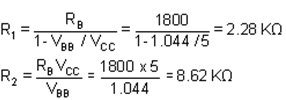The maximum output voltage swing, ignoring the non-linearity's at saturation and cutoff, would be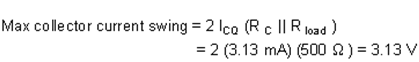The load lines are shown on the characteristics of fig. 6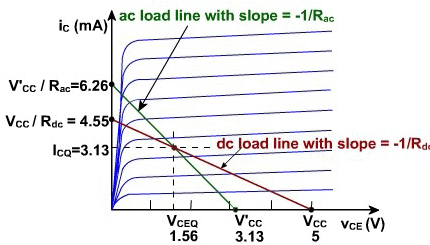Fig. 6
The maximum power dissipated by the transistor is calculated to assure that it does not exceed the specifications. The maximum average power dissipated in the transistor is

P(transistor)= VCEQ ICQ = (1.56 (V)) (3.13 mA) =4.87 mW

This is well within the 350 mW maximum (given on the specification sheet). The maximum conversion efficiency is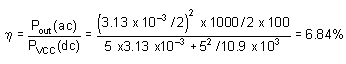The swamped Amplifier:

The ac resistance of the emitter diode r'e equals 25mV /IE and depends on the temperature. Any change in r'e will change the voltage gain in CE amplifier. In some applications, a change in voltage is acceptable. But in many applications we need a stable voltage gain is required.
To make it stable, a resistance rE is inserted in series with the emitter and therefore emitter is no longer ac grounded.(refer to fig .7).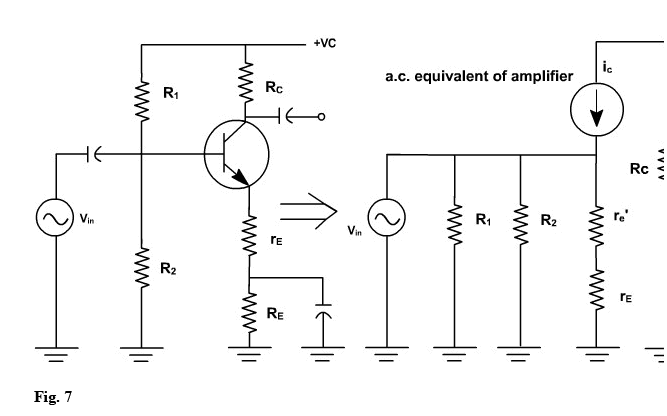Because of this the ac emitter current flows through rE and produces an ac voltage at the emitter.
If rE is much greater than r'e, almost all of the ac input signal appears at the emitter, and the emitter is bootstrapped to the base for ac as well as for dc.
In this case, the collector circuit is given by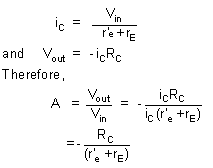Now r'e has a less effect on voltage gain, swamping means rE >> r'e. If swamping is less, voltage gain varies with temperature. If swamping is heavy, then gain reduces significantly.

Offer running on EduRev: Apply code STAYHOME200 to get INR 200 off on our premium plan EduRev Infinity!

,

,

,

,

,

,

,

,

,

,

,

,

,

,

,

,

,

,

,

,

,

;